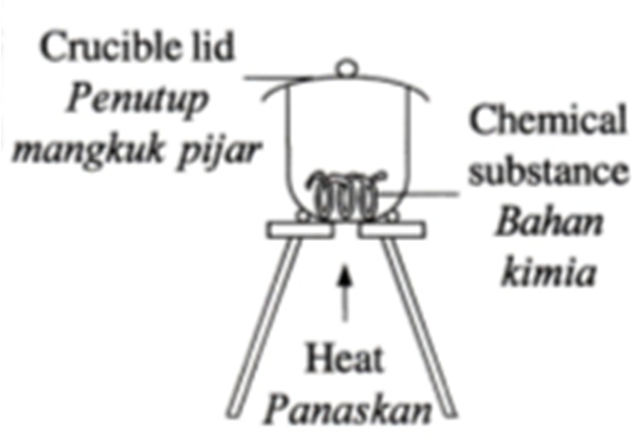## Friday, October 28, 2011

### EXPERIMENT : EMPIRICAL FORMULA (SPOT)

Empirical Formula (Formula Empirik)
Formula show simplest ratio of atoms of each element in the chemical compound.
Formula yang menunjukkan nisbah terkecil atom bagi setiap element di dalam sebatian kimia.

Tips SPM : Calon mesti tahu definisi 'empirical formula'.

ANALISIS SOALAN SPM (2003-2010)

STRUCTURE QUESTION
SPM 2003 /PAPER 2/ QUESTION 1
Experiment to determine the empirical formula of magnesium oxide.
SPM 2007 /PAPER 2/ QUESTION 3
Experiment to determine the empirical formula of two compounds by different methods.
SPM 2009 /PAPER 2/ QUESTION 3
Experiment to determine the empirical formula of copper oxide
SPM 2004/ PAPER3/ QUESTION 1
Experiment to determine the empirical formula of magnesium oxide.

ESSAY QUESTION.
SPM 2010 / PAPER2/ QUESTION 7
Determine the empirical formula and molecular formula of hydrocarbon
CALON MESTI TAHU!!

To determine empirical formula of compounds - two methods used. (For SPM)

#1 - Heat metal in crucible and lids - More reactive metal than hydrogen#2 - Heat metal oxide in excess hydrogen gas in combustion tube and porcelain dish